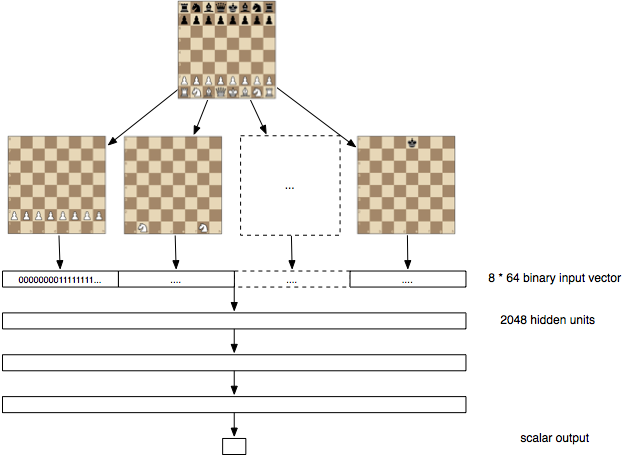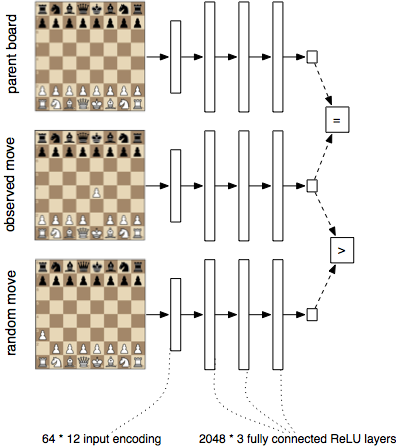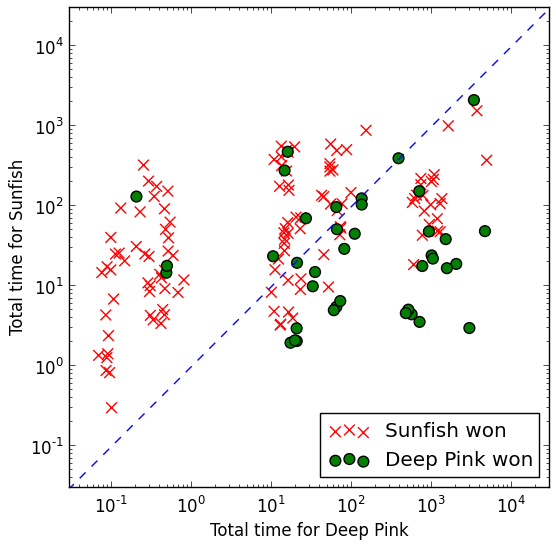# Deep learning for... chess

I've been meaning to learn Theano for a while and I've also wanted to build a chess AI at some point. So why not combine the two? That's what I thought, and I ended up spending way too much time on it. I actually built most of this back in September but not until Thanksgiving did I have the time to write a blog post about it.

What's the theory?

Chess is a game with a finite number of states, meaning if you had infinite computing capacity, you could actually solve chess. Every position in chess is either a win for white, a win for black, or a forced draw for both players. We can denote this by the function $$f(\mbox{position})$$ . If we had an infinitely fast machine we could compute this by

1. Assign all the final positions the value $${-1, 0, 1}$$ depending on who wins.
2. Use the recursive rule

$$f(p) = \max_{p \rightarrow p’} -f(p’)$$

where $$p \rightarrow p’$$ denotes all the legal moves from position $$p$$ . The minus sign is because the players alternate between positions, so if position $$p$$ is white's turn, then position $$p’$$ is black turns (and vice versa). This is the same thing as minimax.

There's approximately $$10^{43}$$ positions, so there's no way we can compute this. We need to resort to approximations to $$f(p)$$ .

What's the point of using machine learning for this?

What machine learning really boils down to is approximating functions given data. So assuming we can get a lot of data to learn this from, we can learn this function $$f(p)$$ . Once we have a model, an objective, and training data, we can go knock ourselves out.

I downloaded 100M games from FICS Games Database and began training a machine learning model. My function $$f(p)$$ is learned from data by using two principles

1. Players will choose an optimal or near-optimal move. This means that for two position in succession $$p \rightarrow q$$ observed in the game, we will have $$f(p) = -f(q)$$ .
2. For the same reason above, going from $$p$$ , not to $$q$$ , but to a random position $$p \rightarrow r$$ , we must have $$f(r) > f(q)$$ because the random position is better for the next player and worse for the player that made the move.

The model

We construct $$f(p)$$ as a 3 layer deep 2048 units wide artificial neural network, with rectified linear units in each layer. The input is a 8 * 8 * 12 = 768 wide layer which indicates whether each piece (there are 12 types) is present in each square (there are 8 * 8 squares). After three matrix multiplications (each followed by a nonlinearity), there's a final dot product with a 2048-wide vector to condense it down to a single value.In total there's roughly 10M unknown parameters in the network.

To train the network, I present it with $$(p, q, r)$$ triplets. I feed it through the network. Denoting by $$S(x) = 1 / (1 + exp(-x))$$ , the sigmoid function, the total objective is:

$$sum_{(p, q, r)} \log S(f(q) - f(r)) + \kappa \log (f(p) + f(q)) + \kappa \log (-f(q) - f(p))$$

This is the log likelihood of the “soft” inequalities $$f(r) > f(q)$$ , $$f(p) > -f(q)$$ , and $$f(p) < -f(q)$$ . The last two are just a way of expressing a “soft” equality $$f(p) = -f(q)$$ . I also use $$\kappa$$ to put more emphasis on getting the equality right. I set it to 10.0. I don't think the solution is super sensitive to the value of $$\kappa$$ .Notice that the function we learn has no idea about the rules of chess. We're not even teaching it how each piece move. We make sure the model has the expressiveness to work out legal moves, but we don't encode any information about the game itself. The model learns this information by observing tons of chess games.

Note that I'm also not trying to learn anything from who won the game. The reason is that the training data is full of games played by amateurs. If a grandmaster came into the middle of a game, s/he could probably completely turn it around. This means the final score is a pretty weak label. Still, even an amateur player probably makes near-optimal moves for most time.

Training the model

I rented a GPU instance from AWS and trained it on 100M games for about four days using stochastic gradient descent with Nesterov momentum. I put all (p, q, r) triplets into a HDF5 data file. I was messing around with learning rates for a while but after a while I realized I just wanted something that would give me good results in a few days. So I ended using a slightly unorthodox learning rate scheme: $$0.03 \cdot \exp(-\mbox{time in days})$$ . Since I had so much training data, regularization wasn't necessary, so I wasn't using either dropout or L2 regularization.

A trick I did was to encode the boards as 64 bytes and then transform the board into a 768 units wide float vector on the GPU. This gave a pretty substantial performance boost since there's a lot less I/O.

How does a chess AI work?

Every chess AI starts with some function $$f(p)$$ that approximates the value of the position. This is known as evaluation function.

This function is also combined with a deep search of many millions of positions down the game tree. It turns out that an approximation of $$f(p)$$ is just a small part of the playing chess well. All chess AI's focus on smart search algorithms, but the number of positions explode exponentially down the search tree, so in practice you can't go deeper than say 5-10 positions ahead. What you do is you use some approximation to evaluate leaf nodes and then use some variety of negamax to evaluate a game tree of a bunch of possible next moves.

By applying some smart searching algorithm, we can take pretty much any approximation and make it better. Chess AI's typically start with some simple evaluation function like: every pawn is worth 1 point, every knight is worth 3 points, etc.

We're going to take the function we learned and use it to evaluate leaves in the game tree. Then try to search deep. So we're first going to learn the function $$f(p)$$ from data, then we're going to plug it into a search algorithm.

Does it work?

I coined my chess engine Deep Pink as an homage to Deep Blue. As it turns out, the function we learn can definitely play chess. It beats me, every time. But I'm a horrible chess player.

Does Deep Pink beat existing chess AI's? Sometimes

I pit it against another chess engine: Sunfish by Thomas Dybdahl Ahle. Sunfish is written entirely in Python. The reason I chose to stick to the same language was that I didn't want this to be an endless exercise of making fast move generation. Deep Pink also relies heavily on quick move generation, and I didn't want to spend weeks working out edge cases with bitmaps in C++ to be able to compete with the state of the art engines. That would just be an arms race. So to be able to establish something useful, I picked a pure Python engine.

The obvious thing in hindsight is: the main thing you want out of any evaluation function $$f(p)$$ isn't accuracy, it's accuracy per time unit. It doesn't matter that one evaluation function is slightly better than another if it's ten times slower, because you can take the fast (but slightly worse) evaluation function and search more nodes in the game tree. So you really want to take into account the time spent by the engine. Without further ado, here's some results of playing against the engine many times:Notice the log-scale. The x-axis and y-axis aren't super relevant here, the main thing is the distance to the diagonal, because that tells us which engine spent more CPU time. Every game I randomized the parameters for each engine: the max depth for Deep Pink, and the max number of nodes for Sunfish. (I didn't include draws because both engines struggle with it).

Not surprisingly, the more time advantage either side has, the better it plays. Overall, Sunfish is better, winning the majority of the games, but Deep Pink probably wins 1/3 of the time. I'm actually pretty encouraged by this. I think with some optimizations, Deep Pink could actually play substantially better:

• Better search algorithm. I'm currently using Negamax with alpha-beta pruning, whereas Sunfish uses MTD-f
• Better evaluation function. Deep Pink plays pretty aggressively, but makes a lot of dumb mistakes. By generating “harder” training examples (ideally fed from mistakes it made) it should learn a better model
• Faster evaluation function: It might be possible to train a smaller (but maybe deeper) version of the same neural network
• Faster evaluation function: I didn't use the GPU for playing, only for training.

Obviously the real goal wouldn't be to beat Sunfish, but one of the “real” chess engines out there. But for that, I would have to write carefully tuned C++ code, and I'm not sure it's the best way to spend my time.

Summary

I'm encouraged by this. I think it's really cool that

1. It's possible to learn an evaluation function directly from raw data, with no preprocessing
2. A fairly slow evaluation function (several orders of magnitude slower) can still play well if it's more accurate
I'm pretty curious to see if this could fare well for Go or other games where AI's still don't play well. Either way, the conclusions above come with a million caveats. The biggest one is obviously that I haven't challenged a “real” chess engine. I'm not sure if I have the time to start hacking on chess engines, but if anyone is interested, I've put all the source code up on Github.

Tagged with: math